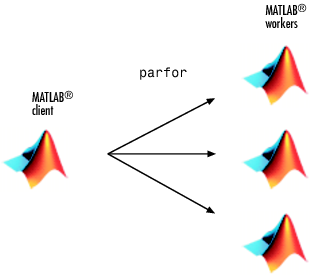## Interactively Run a Loop in Parallel Using `parfor`

In this example, you start with a slow `for`-loop, and you speed up the calculation using a `parfor`-loop instead. `parfor` splits the execution of `for`-loop iterations over the workers in a parallel pool.This example calculates the spectral radius of a matrix and converts a `for`-loop into a `parfor`-loop. Find out how to measure the resulting speedup.

1. In the MATLAB® Editor, enter the following `for`-loop. Add `tic` and `toc` to measure the time elapsed.

```tic n = 200; A = 500; a = zeros(n); for i = 1:n a(i) = max(abs(eig(rand(A)))); end toc```
2. Run the script, and note the elapsed time.

`Elapsed time is 31.935373 seconds.`

3. In the script, replace the `for`-loop with a `parfor`-loop.

```tic n = 200; A = 500; a = zeros(n); parfor i = 1:n a(i) = max(abs(eig(rand(A)))); end toc```

4. Run the new script, and run it again. Note that the first run is slower than the second run, because the parallel pool takes some time to start and make the code available to the workers. Note the elapsed time for the second run.

By default, MATLAB automatically opens a parallel pool of workers on your local machine.

```Starting parallel pool (parpool) using the 'local' profile ... connected to 4 workers. ... Elapsed time is 10.760068 seconds. ```
The `parfor` run on four workers is about three times faster than the corresponding `for`-loop run. The speed-up is smaller than the ideal speed-up of a factor of four on four workers. This is due to parallel overhead, including the time required to transfer data from the client to the workers and back. This example shows a good speed-up with relatively small parallel overhead, and benefits from conversion into a `parfor`-loop. Not all `for`-loop iterations can be turned into faster `parfor`-loops. To learn more, see Decide When to Use parfor.

One key requirement for using `parfor`-loops is that the individual iterations must be independent. Independent problems suitable for `parfor` processing include Monte Carlo simulations and parameter sweeps. For next steps, see Convert for-Loops Into parfor-Loops.

In this example, you managed to speed up the calculation by converting the `for`-loop into a `parfor`-loop on four workers. You might reduce the elapsed time further by increasing the number of workers in your parallel pool, see Scale Up parfor-Loops to Cluster and Cloud.

You can modify your cluster profiles to control how many workers run your loops, and whether the workers are local or on a cluster. For more information on profiles, see Discover Clusters and Use Cluster Profiles.

Modify your parallel preferences to control whether a parallel pool is created automatically, and how long it remains available before timing out. For more information on preferences, see Specify Your Parallel Preferences.

You can run Simulink® models in parallel with the `parsim` command instead of using `parfor`-loops. For more information and examples of using Simulink in parallel, see Run Multiple Simulations (Simulink).